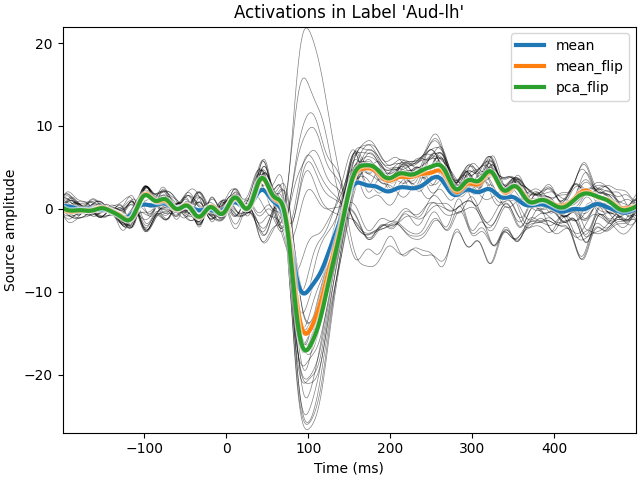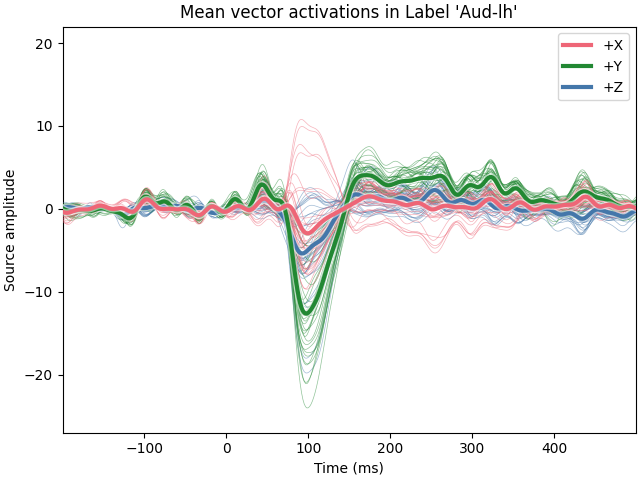# Extracting the time series of activations in a label#

We first apply a dSPM inverse operator to get signed activations in a label (with positive and negative values) and we then compare different strategies to average the times series in a label. We compare a simple average, with an averaging using the dipoles normal (flip mode) and then a PCA, also using a sign flip.

```# Authors: Alexandre Gramfort <alexandre.gramfort@inria.fr>
#          Eric Larson <larson.eric.d@gmail.com>
#
```
```import matplotlib.pyplot as plt
import matplotlib.patheffects as path_effects

import mne
from mne.datasets import sample

print(__doc__)

data_path = sample.data_path()
label = 'Aud-lh'
meg_path = data_path / 'MEG' / 'sample'
label_fname = meg_path / 'labels' / f'{label}.label'
fname_inv = meg_path / 'sample_audvis-meg-oct-6-meg-inv.fif'
fname_evoked = meg_path / 'sample_audvis-ave.fif'

snr = 3.0
lambda2 = 1.0 / snr ** 2
method = "dSPM"  # use dSPM method (could also be MNE or sLORETA)

evoked = mne.read_evokeds(fname_evoked, condition=0, baseline=(None, 0))
src = inverse_operator['src']
```
```Reading /home/circleci/mne_data/MNE-sample-data/MEG/sample/sample_audvis-ave.fif ...
Read a total of 4 projection items:
PCA-v1 (1 x 102) active
PCA-v2 (1 x 102) active
PCA-v3 (1 x 102) active
Average EEG reference (1 x 60) active
Found the data of interest:
t =    -199.80 ...     499.49 ms (Left Auditory)
0 CTF compensation matrices available
nave = 55 - aspect type = 100
Projections have already been applied. Setting proj attribute to True.
Applying baseline correction (mode: mean)
Reading inverse operator decomposition from /home/circleci/mne_data/MNE-sample-data/MEG/sample/sample_audvis-meg-oct-6-meg-inv.fif...
[done]
[done]
305 x 305 full covariance (kind = 1) found.
Read a total of 4 projection items:
PCA-v1 (1 x 102) active
PCA-v2 (1 x 102) active
PCA-v3 (1 x 102) active
Average EEG reference (1 x 60) active
22494 x 22494 diagonal covariance (kind = 2) found.
22494 x 22494 diagonal covariance (kind = 6) found.
22494 x 22494 diagonal covariance (kind = 5) found.
Did not find the desired covariance matrix (kind = 3)
Computing patch statistics...
[done]
Computing patch statistics...
[done]
Read a total of 4 projection items:
PCA-v1 (1 x 102) active
PCA-v2 (1 x 102) active
PCA-v3 (1 x 102) active
Average EEG reference (1 x 60) active
Source spaces transformed to the inverse solution coordinate frame
```

## Compute inverse solution#

```pick_ori = "normal"  # Get signed values to see the effect of sign flip
stc = apply_inverse(evoked, inverse_operator, lambda2, method,
pick_ori=pick_ori)

stc_label = stc.in_label(label)
modes = ('mean', 'mean_flip', 'pca_flip')
tcs = dict()
for mode in modes:
tcs[mode] = stc.extract_label_time_course(label, src, mode=mode)
print("Number of vertices : %d" % len(stc_label.data))
```
```Preparing the inverse operator for use...
Scaled noise and source covariance from nave = 1 to nave = 55
Created the regularized inverter
Created an SSP operator (subspace dimension = 3)
Created the whitener using a noise covariance matrix with rank 302 (3 small eigenvalues omitted)
Computing noise-normalization factors (dSPM)...
[done]
Applying inverse operator to "Left Auditory"...
Picked 305 channels from the data
Computing inverse...
Eigenleads need to be weighted ...
Computing residual...
Explained  59.4% variance
dSPM...
[done]
Extracting time courses for 1 labels (mode: mean)
Extracting time courses for 1 labels (mode: mean_flip)
Extracting time courses for 1 labels (mode: pca_flip)
Number of vertices : 33
```

## View source activations#

```fig, ax = plt.subplots(1)
t = 1e3 * stc_label.times
ax.plot(t, stc_label.data.T, 'k', linewidth=0.5, alpha=0.5)
pe = [path_effects.Stroke(linewidth=5, foreground='w', alpha=0.5),
path_effects.Normal()]
for mode, tc in tcs.items():
ax.plot(t, tc, linewidth=3, label=str(mode), path_effects=pe)
xlim = t[[0, -1]]
ylim = [-27, 22]
ax.legend(loc='upper right')
ax.set(xlabel='Time (ms)', ylabel='Source amplitude',
title='Activations in Label %r' % (label.name),
xlim=xlim, ylim=ylim)
mne.viz.tight_layout()
```## Using vector solutions#

It’s also possible to compute label time courses for a `mne.VectorSourceEstimate`, but only with `mode='mean'`.

```pick_ori = 'vector'
stc_vec = apply_inverse(evoked, inverse_operator, lambda2, method,
pick_ori=pick_ori)
data = stc_vec.extract_label_time_course(label, src)
fig, ax = plt.subplots(1)
stc_vec_label = stc_vec.in_label(label)
colors = ['#EE6677', '#228833', '#4477AA']
for ii, name in enumerate('XYZ'):
color = colors[ii]
ax.plot(t, stc_vec_label.data[:, ii].T, color=color, lw=0.5, alpha=0.5,
zorder=5 - ii)
ax.plot(t, data[0, ii], lw=3, color=color, label='+' + name, zorder=8 - ii,
path_effects=pe)
ax.legend(loc='upper right')
ax.set(xlabel='Time (ms)', ylabel='Source amplitude',
title='Mean vector activations in Label %r' % (label.name,),
xlim=xlim, ylim=ylim)
mne.viz.tight_layout()
``````Preparing the inverse operator for use...
Scaled noise and source covariance from nave = 1 to nave = 55
Created the regularized inverter
Created an SSP operator (subspace dimension = 3)
Created the whitener using a noise covariance matrix with rank 302 (3 small eigenvalues omitted)
Computing noise-normalization factors (dSPM)...
[done]
Applying inverse operator to "Left Auditory"...
Picked 305 channels from the data
Computing inverse...
Eigenleads need to be weighted ...
Computing residual...
Explained  59.4% variance
dSPM...
[done]
Extracting time courses for 1 labels (mode: mean)
```

Total running time of the script: ( 0 minutes 6.740 seconds)

Estimated memory usage: 81 MB

Gallery generated by Sphinx-Gallery